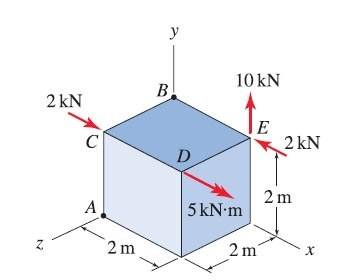# Easy 3d Moment with Couples question, Scalar approach.

• Willjeezy
In summary, Voko explains how to find the moment of a force using the scalar and vector approaches. M = fd and Mx = 5. However, the resultant moments have different orientations, so you will have to take that into account when you sum them up.f

## Homework StatementI'd like to figure out the moment at pt A using the scalar approach, not vector

Vector
M = r x f

Scalar
M = fd

## The Attempt at a Solution

I think I might be missing some concept that would make my life easier...

I figured out how to do this by vector. But I am confused as how to do this by scalar.

for the couple at the top,
My = (2)(2) = 4 j

and then there's a moment at d which causes a rotation in the x axis
Mx= 5 i

The 10kN force can be solved if i put a matching 10kN going up and down at pt A, and then the 10kN pointing down at A and the 10kN pointing up at E would be a couple. However, if I do that the moment calculated is not strictly in one axis.

Kind of confused, I have a feeling I am approaching this wrong.

You can compute the moment of each force "scalarly". But the resultant moments will have different orientations, so you will have to take that into account when you sum them up; which pretty much means you will be treating them as vectors, except that you are going to make a bunch of sign errors.

Hey Voko,

how would I go about figuring out the moment effect of 10kN on pt A. I've tried finding the distance from A to the corner right under e.

= √(2^2 + 2^2)
= 2.8

and then with that length am I able to take the moment of the 10kN force?

M = f d
= (10)(2.8)
= 28

but the problem with this moment is that it is in the x z axis.

Yes, the moment of the 10 kN force is not aligned with any axis. It is at the right angle to Y, meaning it is in XZ plane, and it is at the right angle to AE, which makes it "diagonal" in the XZ plane. The latter means you can represent it as a sum of two moments of equal magnitude, aligned with X and Z axes.

You could have obtained that directly from ##(2, 2, -2) \times (0, 10, 0) = 20(-1, 0, -1)##. Much easier, and no hand waving.

right! thank you.